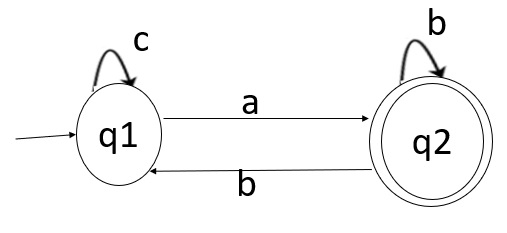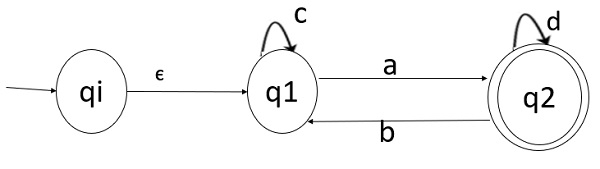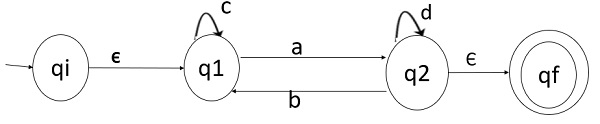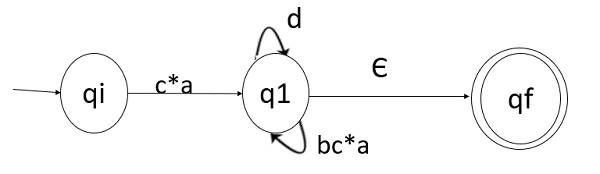# Generate a Regular Expression for a Finite Automata with state elimination method

## Problem

Given a Finite Automata, we need to convert the Finite Automata into equivalent Regular Expression using the State Elimination Method.Step 1 − Initial state q1 has incoming edge. So, create a new initial state qi.Step 2 − Final state q2 has outgoing edge. So, create a new final state.Step 3 − Start eliminating intermediate states one after another.

• If there is path going to qi to q2 via q1
• So after eliminating q1 we can connect a direct path from qi to q2 having cost

ε .c*.a=c*a

• There is a loop on q2 using state qi
• So after eliminating state q1 we can draw a direct loop on state q2 having cost

b.c*.a=b.c*.aStep 4

Now eliminate q2.

After eliminating q2 direct path from state qi to qf having cost.

c*a(d+bc*a) * ε=c*a(d+bc*a)*